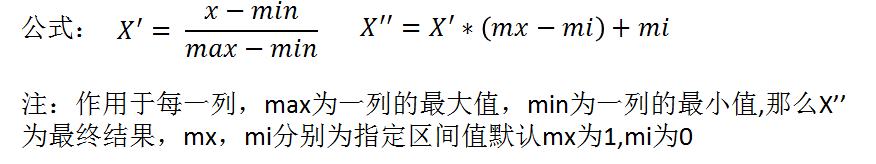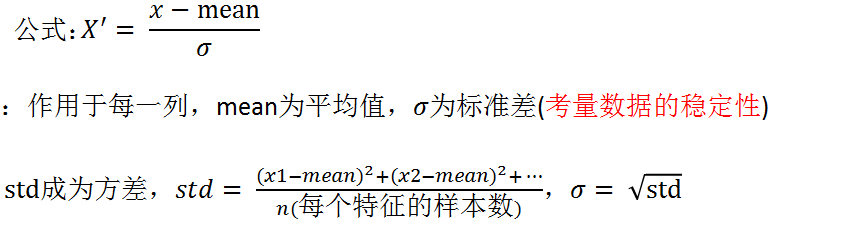# 机器学习-数据处理

1.什么是机器学习

2.数据类型

3.特征工程

4.字典特征抽取

DictVectorizer语法：

DictVectorizer.fit_transform(X), X:字典或者包含字典的迭代器，返回值：返回sparse矩阵（稀疏矩阵）。

DictVectorizer.inverse_transform(X), X:array数组或者sparse矩阵，返回值：转换之前数据格式。

DictVectorizer.get_feature_names( ), 返回类别名称。

DictVectorizer.transform(X), 按照原先的标准转换。

5.文本特征抽取

CountVectorizer语法：

CountVectorizer.fit_transform(X), X:文本或者包含文本字符串的可迭代对象，返回值：返回sparse矩阵

CountVectorizer.inverse_transform(X), X:array数组或者sparse矩阵，返回值：转换之前数据格式

CountVectorizer.get_feature_names( ), 返回值：单词列表

6.TF-IDF

TF-IDF的主要思想是：如果某个词或短语在一篇文章找那个出现的概率高，并且在其他文章中很少出现，则认为                       此词或者短语具有很好的类别区分能力，适合用来分类。

TF-IDF作用：用以评估一字词对于一个文件集或一个语料库中的其中一份文件的重要程度。

TfidfVectorizer语法：

TfidfVectorizer.fit_transform(X),X:文本或者包含文本字符串的可迭代对象，返回值：返回sparse矩阵

TfidfVectorizer.inverse_transform(X),X:array数组或者sparse矩阵，返回值：转换之前数据格式

TfidfVectorizer.get_feature_names( ),返回值：单词列表

7.特征处理

1.归一化   2.标准化   3.缺失值

sklearn特征处理API:sklearn.preprocessingMinMaxScaler语法：

MinMaxScaler.fit_transform(X), X:numpy array 格式的数据[ n_samples, n_features],返回值：转换后的形状                         相同的array归一化和标准化的比较：

StandardScaler语法：

StandardScaler.fit_transform(X), X:numpy array格式的数据[ n_samples, n_features]，返回值：转换后的形状                       相同的array.

StandardScaler.mean_ , 原始数据中每列特征的平均值

StandardScaler.std_ , 原始数据每列特征的方差

sklearn缺失值API:sklearn.preprocessing.Imputer

Imputer语法：

Imputer(missing_values='NaN',strategy='mean',axis = 0)：完成缺失值插补

Imputer.fit_transform(X), X:numpy array格式的数据[ n_samples, n_features ],返回值：转换后的形状相同的                       array

numpy的数组中可以使用np.nan/np.NaN来代替缺失值，属于float类型

8.特征选择

Embedded(嵌入式）：正则化、决策树

Wrapper(包裹式）

VarianceThreshold语法：

VarianceThreshold(threshold = 0.0),删除所有低方差特征

Variance.fit_transform(X), X:numpy array格式的数据[ n_samples, n_features],返回值：训练集差异低于threshold的特征将被删除。默认值是保留所有非零方差特征，即删除所有样本中具有相同值得特征。

sklearn降维API: sklearn.decomposition

9.PCA

PCA:PCA是一种分析、简化数据集的技术。

PCA语法：

PCA( n_components = None ), 将数据分解为较低维数空间

PCA.fit_transform(X),X:numpy array格式的数据[ n_samples, n_features],返回值：转换后制定维度的array.

#encoding=utf-8
from sklearn.feature_extraction import DictVectorizer
from sklearn.feature_extraction.text import CountVectorizer
from sklearn.feature_extraction.text import TfidfVectorizer
from sklearn.preprocessing import MinMaxScaler
from sklearn.preprocessing import StandardScaler
from sklearn.feature_selection import VarianceThreshold
from sklearn.decomposition import PCA
import jieba
"""文本特征提取"""
def dictvec():
#字典数据抽取
dict = DictVectorizer(sparse = False)
#调用fit_transform
data = dict.fit_transform([{'city': '北京','temperature':100}, {'city': '上海','temperature':60}, {'city': '深圳','temperature':30}])
print(dict.get_feature_names())
print(dict.inverse_transform(data))
print(data)
return None
def countvec():
#对文本进行特征值化
cv = CountVectorizer()
data = cv.fit_transform(["life is is short,i like python","life is too long,i dislike python"])
print(cv.get_feature_names())
print(data.toarray())
return None
def cutword():
#jieba分词
con1 = jieba.cut("今天很残酷，明天更残酷，后天很美好，但绝对大部分是死在明天晚上，所以每个人不要放弃今天。")
con2 = jieba.cut("我们看到的从很远星系来的光是在几百万年之前发出的， 这样当我们看到宇宙时，我们是在看它的过去。")
con3 = jieba.cut("如果只用一种方式了解某样事物，你就不会真正了解它。了解事物真正含义的秘密取决于如何将其与我们所了解的事物相联系")

#转换成列表
content1 = list(con1)
content2 = list(con2)
content3 = list(con3)

#把列表转换成字符串
c1 = ' '.join(content1)
c2 = ' '.join(content2)
c3 = ' '.join(content3)

return c1,c2,c3
def hanzivec():
#中文特征值化
c1,c2,c3 = cutword()
print(c1,c2,c3)
cv = CountVectorizer()
data = cv.fit_transform([c1,c2,c3])
print(cv.get_feature_names())
print(data.toarray())

return None
def tfidfvec():
c1,c2,c3 = cutword()
print(c1,c2,c3)
cv = TfidfVectorizer()
data = cv.fit_transform([c1,c2,c3])
print(cv.get_feature_names())
print(data.toarray())
return None
def mm():
#归一化处理
mm = MinMaxScaler(feature_range=(2,3))
#对应的列进行计算
data = mm.fit_transform([[90,2,10,40],[60,4,15,45],[75,3,13,46]])
print(data)
return None

def stand():
#标准化缩放
mm = StandardScaler()
data = mm.fit_transform([[90,2,10,40],[60,4,15,45],[75,3,13,46]])
print(data)
def var():
#特征选择-删除低方差的特征
var = VarianceThreshold(threshold=1.0)
data = var.fit_transform([[0,2,0,3],[0,1,4,3],[0,1,1,3]])
print(data)
return None
def pca():
#主成分分析进行降维
pca = PCA(n_components=0.9)
data = pca.fit_transform([[2,8,4,5],[6,3,0,8],[5,4,9,1]])
print(data)
return None

if __name__=="__main__":
#dictvec()
#countvec()
#tfidfvec()
#mm()
#stand()
#var()
#pca()


©️2019 CSDN 皮肤主题: 大白 设计师: CSDN官方博客